# The Fundamental Identities Fundamental Trigonometric Identities Reciprocal Identities

• Slides: 8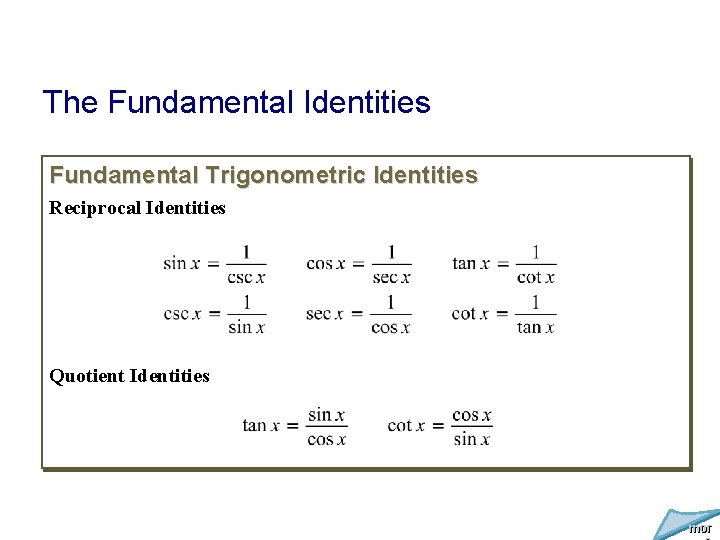The Fundamental Identities Fundamental Trigonometric Identities Reciprocal Identities Quotient Identities mor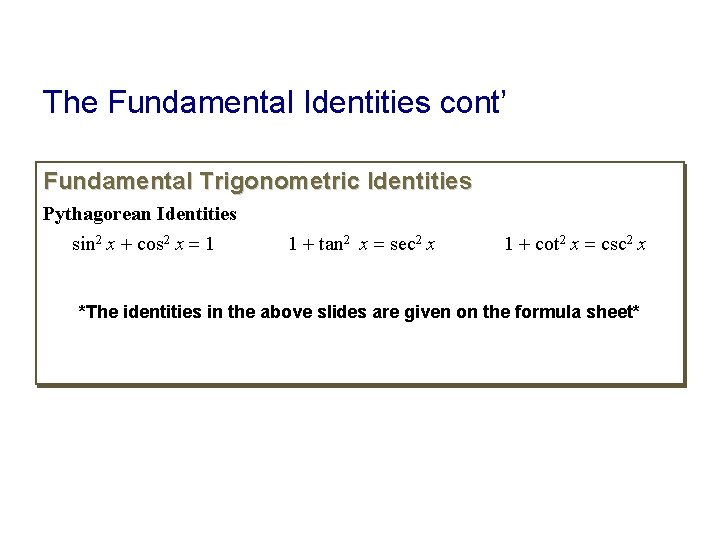The Fundamental Identities cont’ Fundamental Trigonometric Identities Pythagorean Identities sin 2 x + cos 2 x = 1 1 + tan 2 x = sec 2 x 1 + cot 2 x = csc 2 x *The identities in the above slides are given on the formula sheet*Example: Changing to Sines and Cosines to Verify an Identity Verify the identity: sec x cot x = csc x. Solution The left side of the equation contains the more complicated expression. Thus, we work with the left side. Let us express this side of the identity in terms of sines and cosines. Apply a reciprocal identity: sec x = ¹/cos x and a quotient identity: cot x = cos x/sin x. Divide both the numerator and the denominator by cos x, the common factor. Multiply the remaining factors in the numerator and denominator. Apply a reciprocal identity: csc x = ¹/sin x. By working with the left side and simplifying it so that it is identical to the right side, we have verified the given identity. mor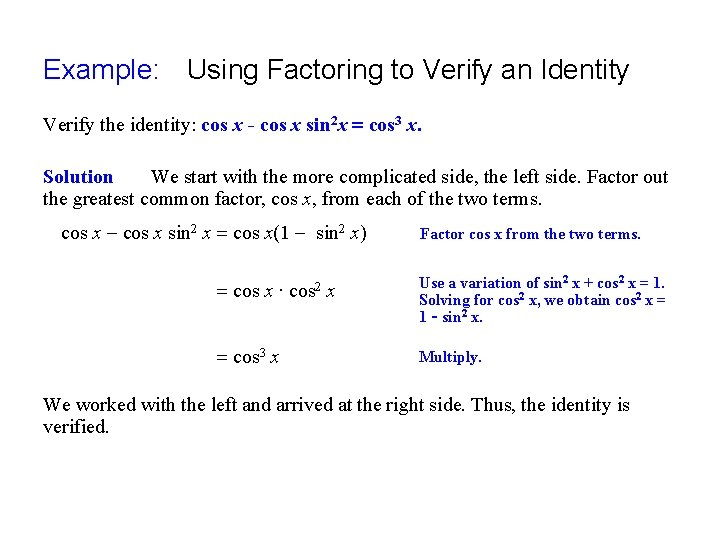Example: Using Factoring to Verify an Identity Verify the identity: cos x - cos x sin 2 x = cos 3 x. Solution We start with the more complicated side, the left side. Factor out the greatest common factor, cos x, from each of the two terms. cos x - cos x sin 2 x = cos x(1 - sin 2 x) = cos x ∙ = cos 3 x cos 2 x Factor cos x from the two terms. Use a variation of sin 2 x + cos 2 x = 1. Solving for cos 2 x, we obtain cos 2 x = 1 – sin 2 x. Multiply. We worked with the left and arrived at the right side. Thus, the identity is verified.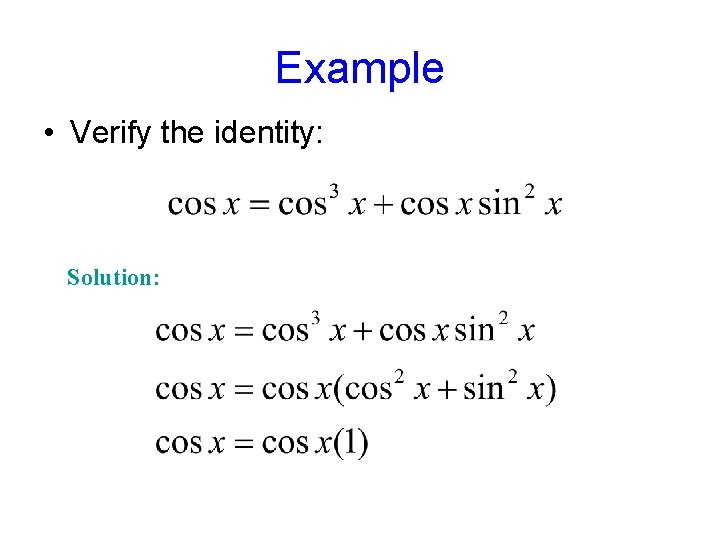Example • Verify the identity: Solution: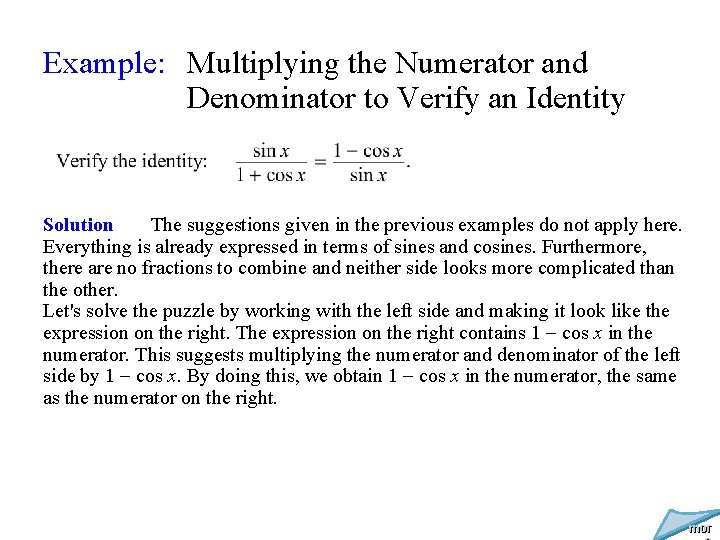Example: Multiplying the Numerator and Denominator to Verify an Identity Solution The suggestions given in the previous examples do not apply here. Everything is already expressed in terms of sines and cosines. Furthermore, there are no fractions to combine and neither side looks more complicated than the other. Let's solve the puzzle by working with the left side and making it look like the expression on the right. The expression on the right contains 1 - cos x in the numerator. This suggests multiplying the numerator and denominator of the left side by 1 - cos x. By doing this, we obtain 1 - cos x in the numerator, the same as the numerator on the right. mor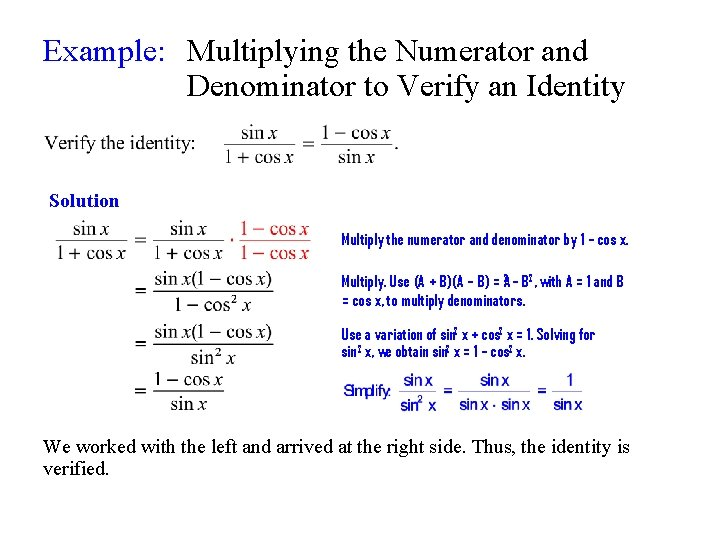Example: Multiplying the Numerator and Denominator to Verify an Identity Solution Multiply the numerator and denominator by 1 – cos x. Multiply. Use (A + B)(A – B) = A 2 – B 2, with A = 1 and B = cos x, to multiply denominators. Use a variation of sin 2 x + cos 2 x = 1. Solving for sin 2 x, we obtain sin 2 x = 1 – cos 2 x. We worked with the left and arrived at the right side. Thus, the identity is verified.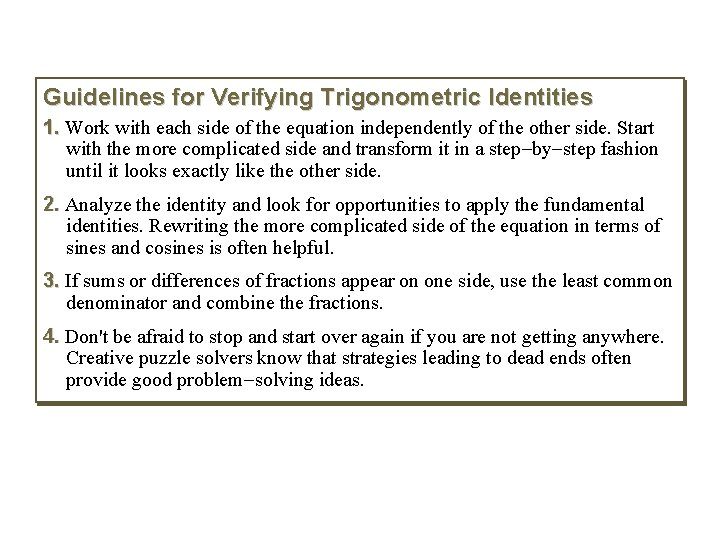Guidelines for Verifying Trigonometric Identities 1. Work with each side of the equation independently of the other side. Start with the more complicated side and transform it in a step-by-step fashion until it looks exactly like the other side. 2. Analyze the identity and look for opportunities to apply the fundamental identities. Rewriting the more complicated side of the equation in terms of sines and cosines is often helpful. 3. If sums or differences of fractions appear on one side, use the least common denominator and combine the fractions. 4. Don't be afraid to stop and start over again if you are not getting anywhere. Creative puzzle solvers know that strategies leading to dead ends often provide good problem-solving ideas.